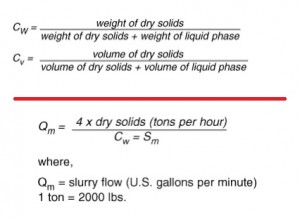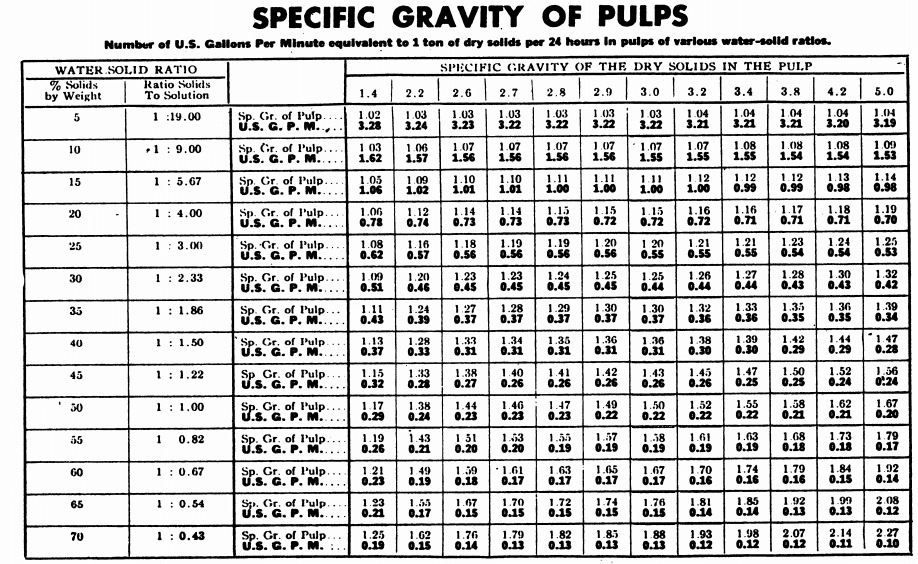# Calculate Slurry Flow / Volume

An example of how you can calculate the slurry flow/volume of a given SG, %Solids and Tonnage.

EXAMPLE: 3,400 tons of dry solids is processed in 24 hours in water with a specific gravity of 1.0 and the
concentration of solids by weight is 33% with the solids having a specific gravity of 3.7 then using the formula below:Calculate the Capacity of an Agitator & Conditioner